JP5510032B2 - Non-contact power feeding device - Google Patents

Info

Publication number
JP5510032B2
JP5510032B2 JP2010101755A JP2010101755A JP5510032B2 JP 5510032 B2 JP5510032 B2 JP 5510032B2 JP 2010101755 A JP2010101755 A JP 2010101755A JP 2010101755 A JP2010101755 A JP 2010101755A JP 5510032 B2 JP5510032 B2 JP 5510032B2
Authority
JP
Japan
Prior art keywords
frequency
capacitor
secondary winding
non
impedance
Prior art date
Legal status (The legal status is an assumption and is not a legal conclusion. Google has not performed a legal analysis and makes no representation as to the accuracy of the status listed.)
Active
Application number
JP2010101755A
Other languages
Japanese (ja)
Other versions
JP2010288441A (en
Inventor

トロンナムチヤイ クライソン

Original Assignee

Priority date (The priority date is an assumption and is not a legal conclusion. Google has not performed a legal analysis and makes no representation as to the accuracy of the date listed.)
Filing date
Publication date
Priority to JP2009117527 priority Critical
Priority to JP2009117527 priority
Application filed by 日産自動車株式会社 filed Critical 日産自動車株式会社
Priority to JP2010101755A priority patent/JP5510032B2/en
Publication of JP2010288441A publication Critical patent/JP2010288441A/en
Application granted granted Critical
Publication of JP5510032B2 publication Critical patent/JP5510032B2/en
Application status is Active legal-status Critical
Anticipated expiration legal-status Critical

Images

•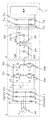•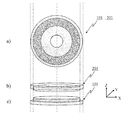•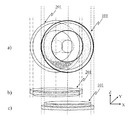•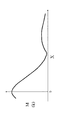•••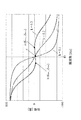•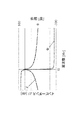•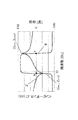•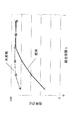•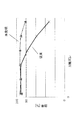•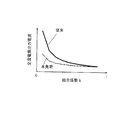•••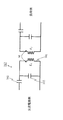••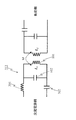•••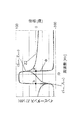•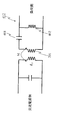•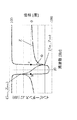•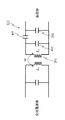•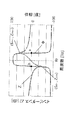•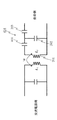••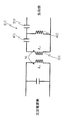•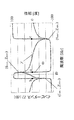•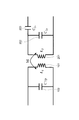•••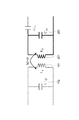••••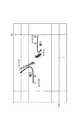•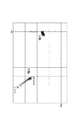•••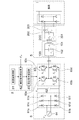•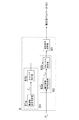••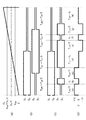•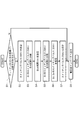••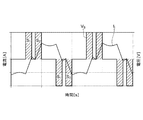•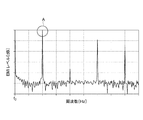•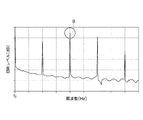•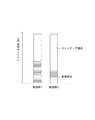••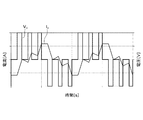•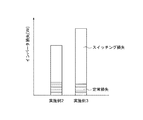•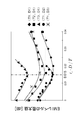•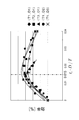Classifications

• HELECTRICITY
• H02GENERATION; CONVERSION OR DISTRIBUTION OF ELECTRIC POWER
• H02JCIRCUIT ARRANGEMENTS OR SYSTEMS FOR SUPPLYING OR DISTRIBUTING ELECTRIC POWER; SYSTEMS FOR STORING ELECTRIC ENERGY
• H02J50/00Circuit arrangements or systems for wireless supply or distribution of electric power
• H02J50/10Circuit arrangements or systems for wireless supply or distribution of electric power using inductive coupling
• HELECTRICITY
• H02GENERATION; CONVERSION OR DISTRIBUTION OF ELECTRIC POWER
• H02JCIRCUIT ARRANGEMENTS OR SYSTEMS FOR SUPPLYING OR DISTRIBUTING ELECTRIC POWER; SYSTEMS FOR STORING ELECTRIC ENERGY
• H02J5/00Circuit arrangements for transfer of electric power between ac networks and dc networks
• H02J5/005Circuit arrangements for transfer of electric power between ac networks and dc networks with inductive power transfer
• HELECTRICITY
• H02GENERATION; CONVERSION OR DISTRIBUTION OF ELECTRIC POWER
• H02JCIRCUIT ARRANGEMENTS OR SYSTEMS FOR SUPPLYING OR DISTRIBUTING ELECTRIC POWER; SYSTEMS FOR STORING ELECTRIC ENERGY
• H02J50/00Circuit arrangements or systems for wireless supply or distribution of electric power
• H02J50/10Circuit arrangements or systems for wireless supply or distribution of electric power using inductive coupling
• H02J50/12Circuit arrangements or systems for wireless supply or distribution of electric power using inductive coupling of the resonant type
• HELECTRICITY
• H02GENERATION; CONVERSION OR DISTRIBUTION OF ELECTRIC POWER
• H02JCIRCUIT ARRANGEMENTS OR SYSTEMS FOR SUPPLYING OR DISTRIBUTING ELECTRIC POWER; SYSTEMS FOR STORING ELECTRIC ENERGY
• H02J50/00Circuit arrangements or systems for wireless supply or distribution of electric power
• H02J50/90Circuit arrangements or systems for wireless supply or distribution of electric power involving detection or optimisation of position, e.g. alignment
• HELECTRICITY
• H02GENERATION; CONVERSION OR DISTRIBUTION OF ELECTRIC POWER
• H02JCIRCUIT ARRANGEMENTS OR SYSTEMS FOR SUPPLYING OR DISTRIBUTING ELECTRIC POWER; SYSTEMS FOR STORING ELECTRIC ENERGY
• H02J7/00Circuit arrangements for charging or depolarising batteries or for supplying loads from batteries
• H02J7/02Circuit arrangements for charging or depolarising batteries or for supplying loads from batteries for charging batteries from ac mains by converters
• H02J7/022Circuit arrangements for charging or depolarising batteries or for supplying loads from batteries for charging batteries from ac mains by converters characterised by the type of converter
• H02J7/025Circuit arrangements for charging or depolarising batteries or for supplying loads from batteries for charging batteries from ac mains by converters characterised by the type of converter using non-contact coupling, e.g. inductive, capacitive
• HELECTRICITY
• H04ELECTRIC COMMUNICATION TECHNIQUE
• H04BTRANSMISSION
• H04B5/00Near-field transmission systems, e.g. inductive loop type
• H04B5/0025Near field system adaptations
• H04B5/0037Near field system adaptations for power transfer

Description

The present invention relates to a non-contact power feeding device.

A series capacitor is connected to the primary winding driven by the AC power supply, a parallel capacitor is connected to the secondary winding, and the value of each capacitor is set based on a predetermined formula to make it approximately equivalent to the ideal transformer. Non-contact power feeding devices are known. (Patent Document 1)

International Publication No. 2007/029438

However, in the conventional non-contact power feeding device, the capacitor is set so as to realize high efficiency on the assumption that the coupling coefficient between the primary winding and the secondary winding is constant. When the voltage changes, there is a problem that the change in the efficiency of the transformer is large.

Therefore, the present invention provides a non-contact power feeding device that reduces changes in the efficiency of a transformer even under conditions where the coupling state changes.

In the present invention, the impedance characteristic for the frequency of Z1 has a maximum value near the frequency of the fundamental wave component of the AC power supply, and the impedance characteristic for the frequency of Z2 is closest to the frequency of the fundamental wave component and takes a maximum value. By having the frequency of the fundamental wave component between the frequency and the frequency that is closest to the frequency of the fundamental wave component and takes a minimum value , the above-described problem is solved.

According to the present invention, since the phase characteristic of the impedance with respect to the frequency viewed from the output side of the AC power supply changes so as to rotate around the fundamental frequency (f 0 ) according to the variation of the coupling coefficient, the coupling coefficient In the case where the impedance is set according to the above, the width of fluctuation of the phase of the impedance is reduced, and as a result, a decrease in efficiency can be suppressed.

It is an electric circuit diagram of the non-contact electric power feeder of this example. FIG. 1 is a plan view and a perspective view of the primary winding and the secondary winding. FIG. 1 is a plan view and a perspective view of the primary winding and the secondary winding. It is a figure which shows the change of the mutual inductance M with respect to the shift | offset | difference of the planar direction of a secondary winding. It is a figure which shows the change of the mutual inductance M with respect to the shift | offset | difference of the secondary winding in the height direction. It is a figure which shows the phase characteristic with respect to the frequency of input impedance (Zin) in the conventional non-contact electric power feeder. In the non-contact electric power feeder of FIG. 1, it is a figure which shows the phase characteristic with respect to the frequency of input impedance (Zin). The contactless power supply device of FIG. 1 is a diagram showing a phase characteristic with respect to frequency of the impedance of only the primary side (Z 1). The contactless power supply device of FIG. 1 is a diagram showing a phase characteristic with respect to frequency of the impedance of only the secondary side (Z 2). It is a characteristic view which shows the electric power feeding efficiency with respect to a coupling coefficient. It is a characteristic view which shows the power feeding efficiency with respect to the shift | offset | difference of a plane direction. It is a characteristic view which shows the output current of the alternating current power supply with respect to a coupling coefficient. It is an electric circuit diagram of the non-contact electric power feeding part which concerns on other embodiment. The contactless power supply apparatus of FIG. 10 is a diagram showing a phase characteristic with respect to frequency of the impedance of only the primary side (Z 1). It is an electric circuit diagram of the non-contact electric power feeding part which concerns on other embodiment. The contactless power supply device of FIG. 12 is a diagram showing a phase characteristic with respect to frequency of the impedance of only the primary side (Z 1). It is an electric circuit diagram of the non-contact electric power feeding part which concerns on other embodiment. The contactless power supply device of FIG. 14 is a diagram showing a phase characteristic with respect to frequency of the impedance of only the primary side (Z 1). It is an electric circuit diagram of the non-contact electric power feeding part which concerns on other embodiment. The contactless power supply device of FIG. 16 is a diagram showing a phase characteristic with respect to frequency of the impedance of only the secondary side (Z 2). It is an electric circuit diagram of the non-contact electric power feeding part which concerns on other embodiment. The contactless power supply device of FIG. 18 is a diagram showing a phase characteristic with respect to frequency of the impedance of only the secondary side (Z 2). It is an electric circuit diagram of the non-contact electric power feeding part which concerns on other embodiment. The contactless power supply device of FIG. 20 is a diagram showing a phase characteristic with respect to frequency of the impedance of only the secondary side (Z 2). It is an electric circuit diagram of the non-contact electric power feeding part which concerns on other embodiment. The contactless power supply device of FIG. 22 is a diagram showing a phase characteristic with respect to frequency of the impedance of only the secondary side (Z 2). It is an electric circuit diagram of the non-contact electric power feeding part which concerns on other embodiment. The contactless power supply apparatus of FIG. 24 is a diagram showing a phase characteristic with respect to frequency of the impedance of only the secondary side (Z 2). It is a circuit diagram of the non-contact electric power feeder which concerns on other embodiment of invention. FIG. 27 is a circuit diagram of a circuit on the primary side in the circuit of the non-contact power feeding unit in FIG. 26. It is a graph which shows the impedance characteristic and phase characteristic of the circuit of FIG. FIG. 27 is a circuit diagram of a parallel circuit of a secondary winding and a capacitor among the circuits on the secondary side (reception side) of the circuit of the contactless power feeding unit in FIG. 26. It is a graph which shows the impedance characteristic and phase characteristic of the secondary side of the non-contact electric power feeding part of FIG. FIG. 27 is a circuit diagram of a secondary side circuit in the non-contact power feeding section of FIG. 26. It is a circuit diagram of the equivalent circuit of the non-contact electric power feeding part of FIG. In the complex plane is a diagram showing the impedance characteristics of the non-contact power supply unit of FIG. 26 (Z in). It is a figure which shows the impedance characteristic in a complex plane. It is a diagram showing the impedance characteristics of the non-contact power supply unit (Z in) of Figure 26. It is a figure which shows the phase characteristic ((PHI) in ) of the non-contact electric power feeding part of FIG. It is a circuit diagram which shows the non-contact electric power feeder which concerns on the other Example of invention. It is a block diagram of the control part of FIG. FIG. 37 is a diagram illustrating output characteristics of a supply voltage (V in ) with respect to time in the non-contact power feeding unit of FIG. 36. It is a figure which shows the output waveform of a carrier waveform, a switching pulse (SW1), a switching pulse (SW2), and a supply voltage in the control part of FIG. It is a flowchart which shows the control procedure of the control part of FIG. In Example 1, it is a figure which shows the characteristic of the supply voltage and electric current with respect to time. In Example 2, it is a figure which shows the characteristic of the supply voltage and electric current with respect to time. FIG. 6 is a diagram illustrating a characteristic of an EMI level with respect to a frequency in Example 1. It is a figure in Example 2 which shows the characteristic of the EMI level with respect to a frequency. It is a graph which shows each inverter loss of Example 1 and Example 2. FIG. It is a graph which shows the characteristic of the efficiency of Example 1 and Example 2 with respect to a coupling coefficient. In Example 3, it is a figure which shows the characteristic of the supply voltage and electric current with respect to time. It is a graph which shows each inverter loss of Example 2 and Example 3. FIG. FIG. 37 is a graph showing characteristics of the maximum value of the EMI level with respect to the duty ratio (D), the period (T), and the rest period (t b ) in the non-contact power feeding unit of FIG. 36. FIG. 37 is a graph showing efficiency characteristics with respect to a duty ratio (D), a period (T), and a rest period (t b ) in the non-contact power feeding unit of FIG. 36.

Hereinafter, embodiments of the invention will be described with reference to the drawings.

<< First Embodiment >>
As an example of a non-contact power supply circuit device according to an embodiment of the invention, a non-contact power supply device used together with a battery for a vehicle such as an electric vehicle and a power load will be described.

FIG. 1 shows an electric circuit diagram of the non-contact power feeding device. The non-contact power supply device according to the present embodiment is supplied with power by the high-frequency power supply circuit 6, the non-contact power supply unit 10 that performs non-contact power supply of power output from the high-frequency AC power supply circuit 6, and the non-contact power supply unit 10. The load 7 is provided.

The high-frequency power circuit 6 is connected to a three-phase AC power source 64, a three-phase AC power source 64, a rectifier 61 that rectifies the three-phase AC into a DC, and a rectifier 61 that is connected to the rectifier 61 via a smoothing capacitor 62. And a voltage type inverter 63 that reversely converts the power to high frequency power. The rectifier 61 connects the diode 61a and the diode 61b, the diode 61c and the diode 61d, and the diode 61e and the diode 61f in parallel, and connects the output of the three-phase AC power supply 64 to each intermediate connection point. The voltage type inverter 63 connects in parallel a series circuit of a switching element 63b similar to a switching element 63a that connects a diode to a MOSFET power transistor or the like in antiparallel, and a series circuit of a similar switching element 63c and switching element 63d. Then, it is connected to the rectifier 61 through the smoothing capacitor 62. Then, an intermediate connection point between the switching element 63a and the switching element 63b and an intermediate connection point between the switching element 63c and the switching element 63d are connected to the power transmission circuit unit 100 that is the primary side of the non-contact power feeding unit 10, respectively. The voltage type inverter 63 supplies AC power of about several k to 100 kHz to the non-contact power feeding unit 100.

The non-contact power feeding unit 10 includes a power transmission circuit unit 100 that is an input side of a transformer and a power receiving circuit unit 200 that is an output side of the transformer. The power transmission circuit unit 100 includes a primary winding 101 and a capacitor 102 connected in parallel to the primary winding 101, and the power receiving circuit unit 200 is connected in parallel to the secondary winding 201 and the secondary winding 201. And a capacitor 203 connected in series to a parallel circuit of the secondary winding 201 and the capacitor 202.

The load unit 7 includes a rectification unit 71 that rectifies AC power supplied from the non-contact power supply unit 10 into a direct current, and a load 72 that is connected to the rectification unit 71. The rectifying unit 71 connects the diode 71a and the diode 71b, and the diode 71c and the diode 71d in parallel, and connects the output of the power receiving circuit unit 200 to each intermediate connection point. Then, the output of the rectifying unit 71 is connected to the load 72.

Next, the mutual inductance M between the primary winding 101 and the secondary winding 201 when the non-contact power supply circuit device shown in FIG. 1 is provided in a vehicle and a parking lot will be described with reference to FIGS.

In this example, the power receiving circuit unit 200 including the secondary winding 201 and the load unit 7 are provided in a vehicle, for example, and the power transmission circuit unit 100 including the primary winding 101 and the high-frequency AC power supply 6 are provided on the ground side, for example, in a parking lot. In the case of an electric vehicle, the load 72 corresponds to, for example, a secondary battery. The secondary winding 201 is provided, for example, in a vehicle chassis. Then, the driver of the vehicle parks in the parking lot so that the secondary winding 201 is above the primary winding 101, and electric power is supplied from the primary winding 101 to the secondary winding 201, and the load 72 The included secondary battery is charged.

2a and 2b show a plan view and a perspective view of the primary winding 101 and the secondary winding 201. FIG. 2a and 2b, the X axis and the Y axis indicate the planar directions of the primary winding 101 and the secondary winding 201, and the Z axis indicates the height direction. 2A and 2B, a) is a plan view of the primary winding 101 and the secondary winding 201, b) is a perspective view of the secondary winding 201, and c) is a perspective view of the primary winding 101. Indicates. For the sake of explanation, the primary winding 101 and the secondary winding 201 have both the same circular shape. However, in this example, the primary winding 101 and the secondary winding 201 are not necessarily circular. Need not have the same shape.

As shown in FIG. 2a, the vehicle may be parked in the parking lot so that the secondary winding 201 matches the primary winding 101 in the X-axis and Y-axis directions which are planar directions. Therefore, as shown in FIG. 2b, the relative positions of the primary winding 101 and the secondary winding 201 may be shifted in the planar direction. Moreover, since the height of a vehicle changes with kinds of vehicles, the height of the primary winding 101 and the secondary winding 201 also changes with vehicle height.

3A shows the change of the mutual inductance M with respect to the deviation of the secondary winding 201 in the X-axis direction shown in FIG. 2, and FIG. 3B shows the mutual inductance with respect to the deviation of the secondary winding 201 in the Z-axis direction shown in FIG. The change of M is shown. As shown in FIG. 2a, when the center of the primary winding 101 coincides with the center of the secondary winding 201, the leakage magnetic flux between the primary winding 101 and the secondary winding 201 is small, and the X axis of FIG. Corresponds to zero, and the mutual inductance M or the coupling coefficient k increases. On the other hand, as shown in FIG. 2b with respect to FIG. 2a, when the positions of the primary winding 101 and the secondary winding 201 are shifted in the X-axis direction, the leakage flux increases, and as shown in FIG. M or the coupling coefficient k becomes small. Further, when the deviation in the height direction between the primary winding 101 and the secondary winding 201 increases, the mutual inductance M or the coupling coefficient k decreases as shown in FIG. 3b.

By the way, a non-contact power supply device or the like used for charging a cordless household appliance such as an electric toothbrush or a shaving shaver or a portable device has a relative relationship between the primary winding 101 and the secondary winding 201. Therefore, it is not necessary to assume that the mutual inductance M fluctuates as described above. Therefore, on the premise of the fixed mutual inductance M, a capacitor included in the power transmission circuit unit 100 and the power reception circuit unit 200 so that power is efficiently supplied to the power reception circuit unit 200 under a specific coupling coefficient k, The inductor is circuit designed.

FIG. 4 shows the phase of impedance (Zin) as viewed from the output side of the AC power supply in the non-contact supply device shown in Patent Document 1 described above by the difference in coupling coefficient between the primary winding and the secondary winding. It is. Here, f 0 indicates the frequency of the fundamental wave component of the AC power supply (hereinafter referred to as fundamental wave frequency), and when the output of the inverter is connected to the power transmission circuit unit 100 as a switching power supply, for example, the inverter is driven. Depends on the switching frequency of the switching element. In this example, it depends on the switching frequency of the switching elements 63a to 63d.

As shown in FIG. 4, when the coupling coefficient k is 0.1, the phase characteristic of the input impedance is zero near the fundamental frequency (f 0 ), so the power factor of the supplied power is 1, and the power is efficiently Can be supplied to the load. On the other hand, if the coupling coefficient k is changed by shifting the positions of the primary winding 101 and the secondary winding 201 without changing the settings of the capacitors and inductors included in the power transmission circuit unit 100 and the power reception circuit unit 200, the coupling coefficient k is changed. Is 0.2, the phase near the fundamental frequency (f 0 ) is greatly delayed. As a result, the power factor of the supplied power decreases, and the efficiency of power supply deteriorates. Further, when the coupling coefficient k is changed and the coupling coefficient k is 0.3, the phase in the vicinity of the fundamental frequency (f 0 ) is further greatly delayed, the power factor of the supplied power is lowered, and the power supply efficiency is deteriorated. .

That is, if power is input at the position of the primary winding 101 and the secondary winding 201 where the coupling coefficient k is 0.1, the power is efficiently supplied. However, when the positions of the primary winding 101 and the secondary winding 201 are shifted and the coupling coefficient k varies, the power supplied to the secondary side is remarkably reduced, resulting in poor efficiency.

In this example, when the coupling coefficient k between the primary winding 101 and the secondary winding 201 takes a predetermined value, the phase characteristic of the input impedance (Zin) of the non-contact power feeding unit 100 viewed from the high-frequency AC power supply 6 side is Near the frequency (f 0 ) of the fundamental wave component of the high-frequency AC power supply 6, it is parallel to the frequency axis. As shown in FIG. 5, in the contactless power supply device of this example, when the coupling coefficient k is 0.3, the phase characteristic of the input impedance (Zin) is near the frequency axis near the fundamental frequency (f 0 ). Become parallel. In other words, the phase characteristic of the input impedance (Zin) has a step, and has a characteristic of flattening near the fundamental frequency (f 0 ). At this time, since the phase of the input impedance (Zin) is close to zero, the power factor of the supplied power is close to 1, and the non-contact power feeding unit 100 efficiently supplies power to the secondary side. Note that “parallel to the frequency axis” does not need to be strictly parallel to the frequency axis, and includes a slight inclination.

In the contactless power supply device of this example, when the coupling coefficient k is varied and the coupling coefficient k is 0.2, the phase characteristic of the input impedance (Zin) is centered around the fundamental frequency (f 0 ). The phase changes so as to rotate, and the phase near the fundamental frequency (f 0 ) does not change much compared to when the coupling coefficient k is 0.3, and remains close to zero. Furthermore, even if the coupling coefficient k is changed and the coupling coefficient k is set to 0.1, the phase characteristic of the input impedance (Zin) changes so as to rotate around the fundamental frequency (f 0 ), and the fundamental wave The phase near the frequency (f 0 ) does not change much compared to when the coupling coefficient k is 0.2 or 0.3 and remains close to zero.

In addition, the phase characteristic of the input impedance in this example is, in other words, when the coupling coefficient k between the primary winding 101 and the secondary winding takes a predetermined value (when k = 0.3 in FIG. 5). The difference between the maximum value (Z MAX ) and the minimum value (Z MIN ) of the phase characteristic of the input impedance (Zin) of the non-contact power feeding unit 100 takes a value close to zero. Here, especially in the case of a phase characteristic having a plurality of local maximum values, the local maximum value (Z MAX ) indicates a value for a frequency closest to the fundamental frequency (f 0 ). The same applies to the minimum value (Z MIN ). In FIG. 5, the phase has a maximum value (Z MAX ) at the frequency (f MAX ) shown at point a, and the phase has a minimum value (Z MIN ) at the frequency (f MIN ) shown at point b. As shown in FIG. 5, the difference between the maximum value (Z MAX ) and the minimum value (Z MIN ) takes a value close to zero.

Furthermore, the phase characteristic of the input impedance of this example is, in other words, when the coupling coefficient k between the primary winding 101 and the secondary winding takes a predetermined value (when k = 0.3 in FIG. 5), The phase characteristic of the input impedance (Zin) of the contact power feeding unit 100 is a characteristic in which the inflection point is in the vicinity of the fundamental frequency (f 0 ) and the tangent line of the inflection point is parallel to the frequency axis. In FIG. 5, a point C indicates an inflection point and is in the vicinity of the fundamental frequency (f 0 ). And as shown in FIG. 5, the tangent of the point C becomes parallel to a frequency axis. Note that being parallel to the frequency axis does not need to be strictly parallel to the frequency axis, and includes a slight inclination.

In this way, by designing the capacitors and inductors included in the non-contact power feeding unit 10, the non-contact power feeding apparatus of the present example has an input as described above at a predetermined value in the variable coupling coefficient k. The phase characteristic of impedance (Zin) is obtained. Next, an example of a circuit for allowing the phase characteristic of the input impedance (Zin) to have the above characteristics will be described with reference to FIG.

FIG. 6a shows impedance characteristics (Z) and phase characteristics (Φ) with respect to the frequency of the impedance (Z 1 ) on the primary side only as viewed from the high-frequency AC power supply 6 side in the non-contact power feeding unit 10 shown in FIG. FIG. 6B shows the impedance characteristic (Z) and the phase characteristic (Φ) with respect to the frequency of the impedance (Z 2 ) only on the secondary side viewed from the load part 7 side in the non-contact power feeding unit 10 shown in FIG. For the impedance (Z 1 ) on the primary side only and the impedance (Z 2 ) on the secondary side only, the impedance on the primary side only and the secondary side only may be calculated with the mutual inductance M being zero.

As shown in FIG. 6a, the impedance characteristic (Z) of the impedance (Z 1 ) takes a local maximum value near the fundamental frequency (f 0 ). The impedance phase characteristic of (Z 1) (Φ) maintains the vicinity to approximately +90 degrees at the fundamental frequency (f 0), the slope of the phase diverges in the vicinity of the fundamental frequency (f 0), basic It maintains about -90 degrees after exceeding the vicinity of the wave frequency (f 0 ).

As shown in Figure 6b, the impedance impedance characteristic (Z) of (Z 2) is provided with a frequency taking the maximum value (Z MAX) (f MAX) , takes the minimum value (Z MIN) frequency (f MIN) and the In between, it has a fundamental frequency (f 0 ). Here, especially in the case of a phase characteristic having a plurality of local maximum values, the local maximum value (Z MAX ) indicates a value for a frequency closest to the fundamental frequency (f 0 ). The same applies to the minimum value (Z MIN ). The phase characteristic (Φ) of the impedance (Z 2 ) has two points (point a and point b shown in FIG. 6b) where the phase gradient diverges, and in parallel with the frequency axis between the two points. And the fundamental frequency component (f 0 ) is between the two points. Alternatively, the phase characteristic (Φ) has a characteristic of returning around the fundamental frequency (f 0 ).

Then, by setting the impedance having the characteristic shown in FIG. 6a in the power transmission circuit unit 100 and setting the impedance having the characteristic shown in FIG. 6b in the power receiving circuit unit 200, as shown in FIG. The power supply unit 100 is set.

As described above, in this example, the positional relationship between the primary winding 101 and the secondary winding 201 varies, and the coupling coefficient k varies, but k = 0.3 in FIG. ), The phase characteristic of the input impedance (Zin) has a flat characteristic with respect to the frequency axis in the vicinity of the fundamental frequency (f 0 ). In other words, in this example, when the coupling coefficient k takes the predetermined value, the phase characteristic of the input impedance (Zin) of the non-contact power feeding unit 100 viewed from the high-frequency AC power supply 6 side is represented by the fundamental wave of the high-frequency AC power supply 6. Near the frequency (f 0 ), it is parallel to the frequency axis. In other words, in the present example, when the coupling coefficient k takes the predetermined value, the difference between the maximum value (Z MAX ) and the minimum value (Z MIN ) of the phase characteristic of the input impedance (Zin) of the non-contact power feeding unit 100. To a value close to zero. Furthermore, in other words, in this example, when the coupling coefficient k takes the predetermined value, the inflection point of the phase characteristic of the input impedance (Zin) of the contact feeding unit 100 is near the fundamental frequency (f 0 ). The tangent of the inflection point is parallel to the frequency axis.

As a result, when the coupling coefficient k varies from the predetermined value, it varies so as to rotate around a point where the phase characteristic of the input impedance (Zin) takes a phase (Φ 0 ) corresponding to the fundamental frequency (f 0 ). Therefore, the fluctuation range of the phase of the input impedance (Zin) with respect to the fundamental frequency (f 0 ) is small, and the fluctuation of the phase (Φ 0 ) can be suppressed even if the coupling coefficient k varies.

In this example, the phase characteristic of the input impedance (Zin) having a predetermined coupling coefficient k takes a phase near zero with respect to the fundamental frequency (f 0 ), and is thus supplied to the power receiving circuit unit 200. When the coupling coefficient k varies from the predetermined value, it is possible to supply power while maintaining high efficiency.

Furthermore, in this example, when the phase characteristic of the input impedance (Zin) having a predetermined coupling coefficient k is changed within a certain range with respect to the predetermined value, the fundamental frequency (f 0 ) Has a characteristic that the phase fluctuates with a value near zero. As a result, even if the coupling coefficient k is varied from a predetermined value within a certain range, this example can maintain a high power factor. As a result, high efficiency can be achieved with respect to the variation of the coupling coefficient k. Electric power can be supplied while maintaining.

For example, when the efficiency of the power supplied by changing the coupling coefficient k varies, the certain range is determined by setting the coupling coefficient k corresponding to the allowable range of the efficiency in advance. The permissible range is appropriately set according to the performance of the primary winding 101 and the secondary winding used, the standard power of the secondary battery serving as a load, and the like.

Hereinafter, the point that the non-contact dielectric device of the present example can maintain higher power supply efficiency than the conventional non-contact dielectric device due to the above-described impedance or phase characteristics will be described with reference to FIGS.

FIG. 7 shows the efficiency of the feeding power with respect to the coupling coefficient k in the contactless power feeding device of this example and the conventional contactless power feeding device. Here, the efficiency (%) shown in FIG. 7 indicates the ratio of the output power output from the non-contact power supply unit 10 to the input power input to the non-contact power supply unit 10. The frequency is the fundamental frequency of the AC power source connected to the input side.

In the conventional non-contact power supply device, the inductor and the capacitor included in the non-contact power supply unit are designed so as to improve the power factor on the premise of the high coupling coefficient k, with emphasis on the power supply efficiency. Therefore, the efficiency is high at the high coupling coefficient k. However, as shown in FIG. 7, when the coupling coefficient k is gradually decreased, the efficiency rapidly deteriorates.

On the other hand, in the non-contact power feeding device of this example, when the coupling coefficient k decreases, it is possible to maintain a higher efficiency state than the conventional example. Also, this example can achieve high efficiency with a small coupling coefficient k.

FIG. 8 shows a change in efficiency when the relative positions of the primary winding 101 and the secondary winding 201 are shifted in the X-axis direction shown in FIG. 2 or FIG. The efficiency is the same as that shown in FIG.

In the conventional non-contact power feeding device, when the relative position of the primary winding 101 and the secondary winding 201 is shifted in the X-axis direction, the coupling coefficient k decreases. Efficiency decreases. On the other hand, in the non-contact power feeding device of this example, even when the relative positions of the primary winding 101 and the secondary winding 201 are shifted, the efficiency can be maintained in a high state. When the efficiency (use conditions) required for the system is 80% and 80% or more is an efficiency that is acceptable in the system, the non-contact power feeding device of this example is The tolerance for reduction in efficiency can be increased by about 1.5 times with respect to the deviation.

FIG. 9 shows a current required on the AC power supply side with respect to the coupling coefficient k when a constant output power needs to be obtained (for example, when a constant power of 10 KW needs to be supplied to the load 72). In the conventional non-contact power supply device, when the coupling coefficient k is high, the power necessary for the power receiving circuit unit 200 can be supplied even if the current flowing through the power transmission circuit unit 100 is small. Since the current flowing through the power transmission circuit unit 100 increases, the loss generated in the primary winding 101 and the like in the circuit increases. On the other hand, when the coupling coefficient k is low, the current flowing through the power transmission circuit unit 100 can be kept small in the contactless power supply device of the present invention, so that power can be efficiently supplied to the power reception circuit unit 200.

In this example, when the coupling coefficient k is 0.3, the primary winding 101, the capacitor, and the capacitor included in the non-contact power feeding unit so that the phase characteristic or impedance characteristic of the input impedance (Zin) has the above characteristic. 102, the secondary winding 201, and the capacitors 202 and 203 are set, but the coupling coefficient k is not necessarily set to 0.3.

That is, under the situation where the position of the secondary winding 201 changes with respect to the position of the primary winding 101, the phase characteristic or impedance characteristic of the input impedance (Zin) is within the assumed fluctuation range of the coupling coefficient k. If the above characteristics are taken, the coupling coefficient k at that time becomes a predetermined value. Furthermore, the efficiency can be improved by designing the circuit so that the phase of the input impedance (Zin) with respect to the fundamental frequency (f 0 ) at this time is close to zero.

Note that the phase characteristic of the impedance (Z 1 ) or the impedance (Z 2 ) may have an extreme value other than the maximum value or the minimum value closest to the fundamental frequency (f 0 ). The high-frequency AC power supply 6 of this example corresponds to the “AC power supply” of the present invention, the capacitor 102 of this example is the “first capacitor” of the present invention, and the capacitor 202 and the capacitor 203 of this example are the present invention. Corresponds to “third capacitor” and “fourth capacitor”.

<< Second Embodiment >>
FIG. 10 is a circuit unit showing a non-contact power feeding apparatus according to another embodiment of the invention. This example is different from the first embodiment described above in that a circuit different from the circuit of the power transmission circuit unit 100 in FIG. 1 is used. Since the other configuration is the same as that of the first embodiment described above, the description thereof is incorporated.

As shown in FIG. 10, the power transmission circuit unit 311 of this example connects the inductor 301 in series with the parallel circuit of the primary winding 101 and the capacitor 202.

FIG. 11 shows the impedance characteristic (Z) and the phase characteristic (Φ) with respect to the frequency of the impedance (Z 1 ) on the primary side only when viewed from the high-frequency AC power supply 6 side, with the mutual inductance M being zero.

As shown in FIG. 11, the impedance characteristic of the impedance of this example (Z 1) has in the vicinity of the fundamental wave frequency (f 0) the maximum value (mountains), a higher frequency than the fundamental wave frequency (f 0) On the other hand, it has a minimum value. Phase characteristic of the addition the impedance (Z 1), the slope of the phase diverges in the vicinity of the fundamental frequency (f 0), at a frequency higher than the fundamental frequency other than the vicinity of the fundamental frequency (f 0) (f 0) Furthermore, it has a point where the phase gradient diverges.

When the non-contact power feeding unit 10 includes the power transmission circuit unit 311 having impedance characteristics or phase characteristics shown in FIG. 11, the phase characteristics of the input impedance (Zin) are as shown in the first embodiment with reference to FIG. Has characteristics. Thereby, in the non-contact power supply device of this example, even if the coupling coefficient k varies, the variation width of the phase of the input impedance (Zin) with respect to the fundamental frequency (f 0 ) is small, and the variation of the phase (Φ 0 ) is small. As a result, the power factor can be maintained at a high value and power can be supplied efficiently.

The inductor 301 of this example corresponds to the “first inductor” of the present invention.

<< Third Embodiment >>
FIG. 12 is a circuit unit showing a non-contact power feeding apparatus according to another embodiment of the invention. This example is different from the first embodiment described above in that a circuit different from the circuit of the power transmission circuit unit 100 in FIG. 1 is used. Since the other configuration is the same as that of the first embodiment described above, the description thereof is incorporated.

As illustrated in FIG. 12, the power transmission circuit unit 312 of this example connects the capacitor 302 in series with the parallel circuit of the primary winding 101 and the capacitor 102.

FIG. 13 shows the impedance characteristic (Z) and phase characteristic (Φ) with respect to the frequency of the impedance (Z 1 ) on the primary side only when viewed from the high-frequency AC power supply 6 side, with the mutual inductance M being zero.

As shown in FIG. 13, the impedance characteristic of the impedance of this example (Z 1) is in the vicinity of the fundamental wave frequency (f 0), have maximum values (peaks), other than in the vicinity of the fundamental wave frequency (f 0) And has a minimum value for a frequency lower than the fundamental frequency (f 0 ). Phase characteristic of the addition the impedance (Z 1), the slope of the phase diverges in the vicinity of the fundamental wave frequency (f 0), at the fundamental wave frequency (f 0) from the low frequency other than the vicinity of the fundamental wave frequency (f 0) Furthermore, it has a point where the phase gradient diverges.

When the non-contact power feeding unit 10 includes the power transmission circuit unit 312 having impedance characteristics or phase characteristics shown in FIG. 13, the phase characteristics of the input impedance (Zin) are as shown in the first embodiment with reference to FIG. Has characteristics. Thereby, in the non-contact power supply device of this example, even if the coupling coefficient k varies, the variation width of the phase of the input impedance (Zin) with respect to the fundamental frequency (f 0 ) is small, and the variation of the phase (Φ 0 ) is small. As a result, the power factor can be maintained at a high value and power can be supplied efficiently.

The capacitor 302 of this example corresponds to the “second capacitor” of the present invention.

<< 4th Embodiment >>
FIG. 14 is a circuit unit showing a non-contact power feeding apparatus according to another embodiment of the invention. This example is different from the first embodiment described above in that a circuit different from the circuit of the power transmission circuit unit 100 in FIG. 1 is used. Since the other configuration is the same as that of the first embodiment described above, the description thereof is incorporated.

As shown in FIG. 14, the power transmission circuit unit 313 of this example connects an inductor 301 to one end of a parallel circuit of a primary winding 101 and a capacitor 102 and a capacitor 302 to the other end of the parallel circuit.

FIG. 15 shows the impedance characteristic (Z) and the phase characteristic (Φ) with respect to the frequency of the impedance (Z 1 ) on the primary side only when viewed from the high-frequency AC power supply 6 side with the mutual inductance M being zero.

As shown in FIG. 15, the impedance characteristic of the impedance of this example (Z 1) is in the vicinity of the fundamental wave frequency (f 0), have maximum values (peaks), other than in the vicinity of the fundamental wave frequency (f 0) It has two minimum values. The phase characteristic of the impedance (Z 1 ) is that the phase gradient diverges near the fundamental frequency (f 0 ), and the phase gradient diverges at frequencies other than the vicinity of the fundamental frequency (f 0 ). There are two points.

When the non-contact power feeding unit 10 includes the power transmission circuit unit 313 having impedance characteristics or phase characteristics shown in FIG. 15, the phase characteristics of the input impedance (Zin) are as shown in the first embodiment with reference to FIG. Has characteristics. Thereby, in the non-contact power supply device of this example, even if the coupling coefficient k varies, the variation width of the phase of the input impedance (Zin) with respect to the fundamental frequency (f 0 ) is small, and the variation of the phase (Φ 0 ) is small. As a result, the power factor can be maintained at a high value and power can be supplied efficiently.

The inductor 301 in this example corresponds to a “first inductor” in the present invention, and the capacitor 302 in the present example corresponds to a “second capacitor” in the present invention.

<< 5th Embodiment >>
FIG. 16 is a circuit unit showing a non-contact power feeding apparatus according to another embodiment of the invention. This example differs from the first embodiment described above in that a circuit different from the circuit of the power receiving circuit unit 200 in FIG. 1 is used. Since the other configuration is the same as that of the first embodiment described above, the description thereof is incorporated.

As shown in FIG. 16, in the power receiving circuit unit 411 of this example, a capacitor 401 is connected in series to the secondary winding 201, and a capacitor 202 is connected in parallel to a series circuit of the secondary winding 201 and the capacitor 401. .

FIG. 17 shows the impedance characteristic (Z) and the phase characteristic (Φ) with respect to the frequency of the impedance (Z 2 ) on the secondary side only when viewed from the load section 7 side, with the mutual inductance M being zero.

As shown in FIG. 17, the impedance impedance characteristic (Z) of (Z 2) is provided with a frequency taking the maximum value (Z MAX) (f MAX) , takes the minimum value (Z MIN) frequency (f MIN) and the In between, it has a fundamental frequency (f 0 ).

The phase characteristic (Φ) of the impedance (Z 2 ) has two points (point a and point b shown in FIG. 17) where the phase gradient diverges, and in parallel with the frequency axis between the two points. And the fundamental frequency component (f 0 ) is between the two points. Alternatively, the phase characteristic (Φ) has a characteristic of returning around the fundamental frequency (f 0 ).

When the power receiving circuit unit 411 having impedance characteristics or phase characteristics shown in FIG. 17 is provided in the non-contact power feeding unit 10, the phase characteristics of the input impedance (Zin) are as shown in the first embodiment with reference to FIG. Has characteristics. Thereby, in the non-contact power supply device of this example, even if the coupling coefficient k varies, the variation width of the phase of the input impedance (Zin) with respect to the fundamental frequency (f 0 ) is small, and the variation of the phase (Φ 0 ) is small. As a result, the power factor can be maintained at a high value and power can be supplied efficiently.

The capacitor 401 in this example corresponds to a “fifth capacitor” in the present invention, and the capacitor 202 in this example corresponds to a “third capacitor” in the present invention.

<< 6th Embodiment >>
FIG. 18 is a circuit unit showing a non-contact power feeding device according to another embodiment of the invention. This example differs from the first embodiment described above in that a circuit different from the circuit of the power receiving circuit unit 200 in FIG. 1 is used. Since the other configuration is the same as that of the first embodiment described above, the description thereof is incorporated.

As shown in FIG. 18, in the power receiving circuit unit 412 of this example, a capacitor 401 is connected in series to the secondary winding 201, and an inductor 402 is connected in parallel to a series circuit of the secondary winding 201 and the capacitor 401. .

FIG. 19 shows the impedance characteristic (Z) and the phase characteristic (Φ) with respect to the frequency of the impedance (Z 2 ) on the secondary side only when viewed from the load section 7 side, with the mutual inductance M being zero.

As shown in FIG. 19, the impedance impedance characteristic (Z) of (Z 2) is provided with a frequency taking the maximum value (Z MAX) (f MAX) , takes the minimum value (Z MIN) frequency (f MIN) and the In between, it has a fundamental frequency (f 0 ). Unlike the impedance characteristic (Z) shown in FIG. 6b, the frequency (f MAX ) is lower than the fundamental frequency (f 0 ), and the frequency (f MIN ) is higher than the fundamental frequency (f 0 ).

The phase characteristic (Φ) of the impedance (Z 2 ) has two points (point a and point b shown in FIG. 19) where the phase gradient diverges, and in parallel with the frequency axis between the two points. And the fundamental frequency component (f 0 ) is between the two points. Alternatively, the phase characteristic (Φ) has a characteristic of returning around the fundamental frequency (f 0 ).

When the power receiving circuit section 412 having impedance characteristics or phase characteristics shown in FIG. 19 is provided in the non-contact power feeding section 10, the phase characteristics of the input impedance (Zin) are as shown in the first embodiment with reference to FIG. Has characteristics. Thereby, in the non-contact power supply device of this example, even if the coupling coefficient k varies, the variation width of the phase of the input impedance (Zin) with respect to the fundamental frequency (f 0 ) is small, and the variation of the phase (Φ 0 ) is small. As a result, the power factor can be maintained at a high value and power can be supplied efficiently.

The capacitor 401 in this example corresponds to a “fifth capacitor” in the present invention, and the inductor 402 in this example corresponds to a “third inductor” in the present invention.

Alternatively, the capacitor 401 and the inductor 402 may be interchanged, the inductor 402 may be connected in series with the secondary winding 201, and the capacitor 402 may be connected to a series circuit of the secondary winding 201 and the inductor 402. At this time, the capacitor 401 of the present example corresponds to a “third capacitor” of the present invention, and the inductor 402 of the present example corresponds to a “fourth inductor” of the present invention.

<< 7th Embodiment >>
FIG. 20 is a circuit unit showing a non-contact power feeding apparatus according to another embodiment of the invention. This example differs from the first embodiment described above in that a circuit different from the circuit of the power receiving circuit unit 200 in FIG. 1 is used. Since the other configuration is the same as that of the first embodiment described above, the description thereof is incorporated.

As shown in FIG. 20, the power receiving circuit unit 413 of this example connects a capacitor 401 in series to the secondary winding 201, a connection point between one end of the secondary winding 201 and the capacitor 401, and the secondary winding. A capacitor 403 is connected between the other end of 201. A capacitor 202 is connected in parallel to a parallel circuit of the secondary winding 201 and the capacitor 403 and a series circuit of the capacitor 401.

FIG. 21 shows the impedance characteristic (Z) and the phase characteristic (Φ) with respect to the frequency of the impedance (Z 2 ) on the secondary side only when viewed from the load section 7 side, with the mutual inductance M being zero.

As shown in FIG. 21, the impedance impedance characteristic (Z) of (Z 2) is provided with a frequency taking the maximum value (Z MAX) (f MAX) , takes the minimum value (Z MIN) frequency (f MIN) and the In between, it has a fundamental frequency (f 0 ).

The phase characteristic (Φ) of the impedance (Z 2 ) has two points (point a and point b shown in FIG. 21) where the phase gradient diverges, and is parallel to the frequency axis between the two points. And the fundamental frequency component (f 0 ) is between the two points. Alternatively, the phase characteristic (Φ) has a characteristic of returning around the fundamental frequency (f 0 ).

When the power receiving circuit unit 413 having impedance characteristics or phase characteristics shown in FIG. 21 is provided in the non-contact power feeding unit 10, the phase characteristics of the input impedance (Zin) are as shown in the first embodiment with reference to FIG. Has characteristics. Thereby, in the non-contact power supply device of this example, even if the coupling coefficient k varies, the variation width of the phase of the input impedance (Zin) with respect to the fundamental frequency (f 0 ) is small, and the variation of the phase (Φ 0 ) is small. As a result, the power factor can be maintained at a high value and power can be supplied efficiently.

The capacitor 403 in this example corresponds to the “sixth capacitor” in the present invention.

<< Eighth Embodiment >>
FIG. 22 is a circuit unit showing a non-contact power feeding apparatus according to another embodiment of the invention. This example differs from the first embodiment described above in that a circuit different from the circuit of the power receiving circuit unit 200 in FIG. 1 is used. Since the other configuration is the same as that of the first embodiment described above, the description thereof is incorporated.

As shown in FIG. 22, the power receiving circuit unit 414 of this example has a capacitor 202 connected in parallel to the series circuit of the secondary winding 201 and the capacitor 401, and a capacitor is connected to the connection point between the capacitor 202 and the capacitor 401. 203 is connected.

FIG. 23 shows the impedance characteristic (Z) and the phase characteristic (Φ) with respect to the frequency of the impedance (Z 2 ) on the secondary side only when viewed from the load section 7 side, with the mutual inductance M being zero.

As shown in FIG. 23, the impedance impedance characteristic (Z) of (Z 2) is provided with a frequency taking the maximum value (Z MAX) (f MAX) , takes the minimum value (Z MIN) frequency (f MIN) and the In between, it has a fundamental frequency (f 0 ). Here, especially in the case of a phase characteristic having a plurality of local maximum values, the local maximum value (Z MAX ) indicates a value for a frequency closest to the fundamental frequency (f 0 ). The same applies to the minimum value (Z MIN ).

The phase characteristic (Φ) of the impedance (Z 2 ) has two points (point a and point b shown in FIG. 23) where the phase gradient diverges, and in parallel with the frequency axis between the two points. And the fundamental frequency component (f 0 ) is between the two points. Alternatively, the phase characteristic (Φ) has a characteristic of returning around the fundamental frequency (f 0 ).

When the power receiving circuit unit 414 having impedance characteristics or phase characteristics shown in FIG. 23 is provided in the non-contact power feeding unit 10, the phase characteristics of the input impedance (Zin) are as shown in the first embodiment with reference to FIG. Has characteristics. Thereby, in the non-contact power supply device of this example, even if the coupling coefficient k varies, the variation width of the phase of the input impedance (Zin) with respect to the fundamental frequency (f 0 ) is small, and the variation of the phase (Φ 0 ) is small. As a result, the power factor can be maintained at a high value and power can be supplied efficiently.

The capacitor 202 in this example corresponds to a “third capacitor”, the capacitor 401 corresponds to a “fifth capacitor”, and the capacitor 203 corresponds to a “fourth capacitor” in the present invention.

<< Ninth Embodiment >>
FIG. 24 is a circuit unit showing a non-contact power feeding apparatus according to another embodiment of the invention. This example differs from the first embodiment described above in that a circuit different from the circuit of the power receiving circuit unit 200 in FIG. 1 is used. Since the other configuration is the same as that of the first embodiment described above, the description thereof is incorporated.

As shown in FIG. 24, the power receiving circuit unit 415 of this example has an inductor 402 connected in parallel to the series circuit of the secondary winding 201 and the capacitor 401, and a capacitor at the connection point between the capacitor 202 and the capacitor 401. 203 is connected.

FIG. 25 shows the impedance characteristic (Z) and the phase characteristic (Φ) with respect to the frequency of the impedance (Z 2 ) on the secondary side only when viewed from the load section 7 side, with the mutual inductance M being zero.

As shown in FIG. 25, the impedance impedance characteristic (Z 2) (Z) is the maximum value and frequency of taking the (Z MAX) (f MAX) , and the frequency taking the minimum value (Z MIN1) (f MIN1) In between, it has a fundamental frequency (f 0 ). In addition to the minimum value (Z MIN1 ), it has a minimum value (Z MIN2 ). Here, the minimum value for the frequency (f MIN1 ) closest to the fundamental frequency (f 0 ) is defined as Z MIN1 .

The phase characteristic (Φ) of the impedance (Z 2 ) has two points (point a and point b shown in FIG. 25) where the phase gradient diverges across the fundamental frequency component (f 0 ). Between the two points, there is a portion parallel to the frequency axis. In addition to the two points where the phase gradient diverges, there is a point (point c shown in FIG. 25) where the phase gradient diverges. Alternatively, the phase characteristic (Φ) has a characteristic of returning around the fundamental frequency (f 0 ).

When the power receiving circuit unit 415 having impedance characteristics or phase characteristics shown in FIG. 25 is provided in the non-contact power feeding unit 10, the phase characteristics of the input impedance (Zin) are as shown in the first embodiment with reference to FIG. Has characteristics. Thereby, in the non-contact power supply device of this example, even if the coupling coefficient k varies, the variation width of the phase of the input impedance (Zin) with respect to the fundamental frequency (f 0 ) is small, and the variation of the phase (Φ 0 ) is small. As a result, the power factor can be maintained at a high value and power can be supplied efficiently.

The capacitor 401 in this example corresponds to a “fifth capacitor”, the capacitor 203 corresponds to a “fourth capacitor”, and the inductor 402 corresponds to a “third inductor” in the present invention.

The power transmission circuit units 100, 311, 312, and 313 and the power reception circuit units 200, 411, 412, 413, 414, and 415 described in the first to ninth embodiments may be arbitrarily combined to form the contactless power feeding unit 10.

<< 10th Embodiment >>
FIG. 26 is a circuit unit showing a non-contact power feeding device according to another embodiment of the invention. This example is different from the first embodiment described above in that the conditions of the inductance of the primary winding 101 and the secondary winding 201 and the capacitance of the capacitors 102, 202, and 203 are defined. Since the other configuration is the same as that of the first embodiment described above, the description thereof is incorporated.

As shown in FIG. 26, a primary winding 101 and a capacitor 102 connected in parallel with the primary winding 101 are provided on the primary side. On the secondary side, a secondary winding 201, a capacitor 202 connected in parallel to the secondary winding, and a parallel circuit of the secondary winding 201 and the capacitor 202 are connected in series. A capacitor 203 is provided. The circuit corresponds to the non-contact power feeding unit 10 shown in FIG. Here, the inductance of the primary winding 101 and L 1, the inductance of the secondary winding 201 L 2, the capacitance C 1p of the capacitor 102, the capacitance of the capacitor 202 C 2p, the electrical capacitance of the capacitor 203 C 2S .

In this example, the conditions of the inductance of the primary winding 101 and the secondary winding 201 and the capacitance of the capacitors 102, 202, and 203 are defined, and the fundamental frequency (f 0 ) is changed to the impedance on the primary side. (Z 1 ) is set near the resonance frequency (f 1 ), and the fundamental frequency (f 0 ) is set between the resonance frequency (fa) of the secondary-side impedance (Z 2 ) and the resonance frequency (fb). To do.

First, the electric capacity C 1p of the capacitor 102 will be described with reference to FIG. FIG. 27 shows a circuit on the primary side (transmission side) in the circuit of FIG.

As shown in FIG. 27, the mutual inductance M = 0 between the primary winding 101 and the secondary winding 201 is set. The circuit design is made so that the relationship between the fundamental frequency (f 0 ) supplied from the high-frequency AC power supply 6 to the primary circuit, the inductance (L 1), and the electric capacity (C 1p ) satisfies Equation 1. Has been.

Next, the impedance characteristic and phase characteristic of the primary side circuit shown in FIG. 27 are shown in FIG. FIG. 28 is a graph showing the impedance characteristic and phase characteristic of the primary-side circuit with respect to frequency.

The resonance frequency (f1) of the impedance (Z 1 ) corresponds to the frequency that shows the maximum value of the impedance characteristic and the frequency of the center point of the rotating phase characteristic. Therefore, it is confirmed from FIG. 28 that the fundamental frequency (f 0 ) is positioned in the vicinity of the resonance frequency (f 1). In other words, the condition of Equation 1 is satisfied by designing the circuit so that the fundamental frequency (f 0 ) is set near the resonance frequency (f 1).

Thereby, the electric current supplied with respect to the non-contact electric power feeding part 10 from the high frequency alternating current power supply 6 can be restrained low, and efficiency can be improved.

Next, the electric capacity C2p of the capacitor 102 will be described with reference to FIG. FIG. 29 shows a parallel circuit of the secondary winding 201 and the capacitor 202 among the secondary side (reception side) circuits of the circuit of FIG.

As shown in FIG. 29, the mutual inductance between the primary winding 101 and the secondary winding 201 is set to zero. The circuit is designed so that the relationship between the inductance (L1) and the capacitance (C 1p ) and the inductance (L2) and the capacitance (C 2p ) satisfies Equation 2.

Equation 2 will be described with reference to FIG. 30 showing the impedance characteristic and phase characteristic of the secondary side circuit of the circuit of FIG. FIG. 30 is a graph showing the impedance characteristic and phase characteristic of the secondary-side circuit with respect to frequency.

As shown in FIG. 30, the resonance frequency (fb) of the impedance (Z 2 ) corresponds to the frequency indicating the maximum value of the impedance characteristic and the frequency of the center point of the rotating phase characteristic. The resonance frequency (fb) is a resonance frequency formed by a resonance circuit (see FIG. 29) with an inductance (L2) and an electric capacity (C 2p ), and between the resonance circuit and the resonance frequency (fb). Have the relationship of (Equation 3).

When the circuit is designed so that the resonance frequency (fb) is lower than the fundamental frequency (f 0 ), (Equation 4) is established.

By substituting Equation 1 and Equation 3 for Equation 4, Equation 2 is derived. That is, the condition of Expression 2 is satisfied by designing the circuit so that the fundamental frequency (f 0 ) is set to a frequency lower than the resonance frequency (fb) so as to satisfy Expression 4.

Next, the electric capacity C 2s of the capacitor 102 will be described with reference to FIG. FIG. 31 shows a circuit on the secondary side (reception side) of the circuit of FIG. 26, and includes a parallel circuit of a secondary winding 201 and a capacitor 202, and a capacitor 203 in which a capacitor 203 is connected in series to the parallel circuit. The circuit which has is shown.

As shown in FIG. 31, it is assumed that the mutual inductance between the primary winding 101 and the secondary winding 201 = 0. The circuit is designed so that the relationship between the inductance (L1) and the capacitance (C 1p ), the inductance (L2), the capacitance (C 2p ), and the capacitance (C 2s ) satisfies Equation 5. .

Expression 5 will be described with reference to FIG. 30 showing the impedance characteristics and phase characteristics of the secondary circuit of the circuit of FIG.

As shown in FIG. 30, the resonance frequency (fa) of the impedance (Z 2 ) corresponds to the frequency indicating the minimum value of the impedance characteristic and the frequency of the center point of the rotating phase characteristic. The resonance frequency (fa) is a resonance frequency of a resonance circuit formed by an inductance (L2), an electric capacity (C 2p ), and an electric capacity (C 2s ), and is between the resonance circuit and the resonance frequency (fa). Has the relationship of (Equation 6).

When the circuit is designed so that the resonance frequency (fa) is higher than the fundamental frequency (f 0 ), (Expression 7) is established.

By substituting Equation 1 and Equation 5 for Equation 7, Equation 6 is derived. That is, the condition of Expression 6 is satisfied by designing the circuit so that the fundamental frequency (f 0 ) is set to a frequency lower than the resonance frequency (fb) so as to satisfy Expression 7.

From Equations 2 and 5, the relationship between the inductance and the capacitance of the primary side circuit and the secondary side circuit. Equation 8 is derived.

Thereby, the phase characteristic of the impedance (Z 2 ) has two points (corresponding to the frequency (fa) and the frequency (fb) shown in FIG. 30) at which the phase gradient diverges, and the two points. And having a portion parallel to the frequency axis, the fundamental frequency component (f 0 ) is between the frequency (fa) and the frequency (fb). As a result, the efficiency of power supplied from the primary side to the secondary side is improved.

Next, the impedance characteristic seen from the output side of the high frequency AC power supply 6 in the circuit of the non-contact power feeding circuit unit 5 shown in FIG. 26 will be described.

FIG. 32 shows an equivalent circuit of the circuit shown in FIG. FIG. 32 shows an equivalent circuit of the circuit of FIG.

Then, based on the circuit shown in FIG. 32, the impedance characteristic (Z in ) viewed from the output side of the high-frequency AC power supply 6 is Laplace transformed, and is shown in Equation 9.

FIG. 33 is a diagram illustrating a polar locus of the impedance characteristic (Z in ). FIG. 33 shows a representative characteristic root having a great influence on the circuit characteristics, that is, the pole 1 closest to the imaginary axis side and the pole 2 closest to the imaginary axis side among the poles of Equation 9. When the coupling coefficient k between the primary winding 101 and the secondary winding 201 is increased from around 0, the pole 1 and the pole 2 draw a locus as shown in FIG. That is, the pole 1 moves away from the imaginary axis as the coupling coefficient increases, and the pole 2 exhibits a characteristic that approaches the first pole as the coupling coefficient increases.

That is, as the coupling coefficient increases, the pole 1 moves away, but the pole 2 approaches the pole 1, so that the influence is canceled out by the mutual pole 1 and pole 2, and as a result, the efficiency reduction is suppressed. It is done. That is, the two poles (pole 1 and pole 2) of the representative characteristic root draw trajectories in opposite directions as k changes.

On the other hand, in the circuit shown in FIG. 26 or FIG. 32, the impedance characteristics viewed from the output side of the high-frequency AC power supply 6 are shown while setting the inductance and capacitance of each circuit so as not to satisfy Equations 1 and 8. , As shown in FIG. FIG. 34 is a diagram illustrating a polar locus of impedance characteristics (Z in ) in a complex plane under circuit conditions that do not satisfy Expressions 1 and 8.

As shown in FIG. 34, the pole 1 closest to the imaginary axis side does not deviate from the imaginary axis as the coupling coefficient increases, and the pole 2 closest to the imaginary axis side increases as the coupling coefficient increases. Keep away from the axis. In addition, as compared with FIG. 33, the pole 1 and the pole 2 draw a locus at a distant position as the coupling coefficient increases (the pole 2 does not become a representative characteristic root), and thus do not affect each other. Therefore, as the coupling coefficient increases, the dominant root moves away from the imaginary axis, and the efficiency decreases.

That is, when there is a pole 1 that moves away from the imaginary axis as the coupling coefficient increases, in the circuit of this example, there are a pole 1 that moves away from the imaginary axis and a pole 2 that approaches the imaginary axis (two representative characteristic roots). The poles (pole 1 and pole 2) are opposite to each other in accordance with the change of k), and the dominant root is switched from pole 1 to pole 2 as the coupling coefficient increases. Therefore, this example has a characteristic that a dominant root exists in the vicinity of the imaginary axis when the coupling coefficient increases. As a result, a change in efficiency associated with a change in coupling coefficient can be suppressed.

Next, impedance characteristics (Z in ) and phase characteristics (Φ in ) viewed from the output side of the high-frequency AC power supply 6 will be described. FIG. 35a shows impedance characteristics (Z in ) in the non-contact power feeding unit 10 of this example, and FIG. 35b shows phase characteristics (Φ in ). FIG. 35a and FIG. 35b show changes in the respective characteristics accompanying changes in the coupling coefficient.

As shown in FIG. 35b, this example has a characteristic that the phase rotates around the vicinity of the fundamental frequency (f 0 ) as the coupling coefficient increases. For this reason, even if the coupling coefficient changes, the phase with respect to the fundamental frequency (f 0 ) takes a value around 0 degrees, so that a decrease in power factor can be suppressed.

As described above, in this example, the primary winding 101 and the capacitor 102 are connected in parallel on the primary side, the parallel circuit of the secondary winding 201 and the capacitor 202 on the secondary side, and the parallel circuit in series. In the non-contact power supply unit 10 to which the capacitor 203 to be connected is connected, the fundamental frequency (f 0 ) of the AC power supplied from the high-frequency AC power source 6 to the non-contact power supply unit 10 is changed to the resonance frequency (Z1) set near the f 1), and is set between the impedance (the resonant frequency of the Z 2) (fa) the impedance (resonance frequency of the Z 2) (fb). Thereby, with the change of the coupling coefficient, the fluctuation of the phase can be suppressed in the vicinity of the fundamental frequency (f 0 ), and the decrease in efficiency can be suppressed.

In this example, the circuit is designed so that the conditions of (Expression 1) and (Expression 8) are satisfied in the above circuit. Thereby, with the change of the coupling coefficient, the fluctuation of the phase can be suppressed in the vicinity of the fundamental frequency (f 0 ), and the decrease in efficiency can be suppressed.

Further, in this example, when the input impedance characteristic (Z in ) is shown in a complex plane in the above circuit, the pole 1 closest to the imaginary axis moves away from the imaginary axis and increases to the imaginary axis as the coupling coefficient increases. The second closest pole 2 approaches the pole 1. Thereby, with the change of the coupling coefficient, the fluctuation of the phase can be suppressed in the vicinity of the fundamental frequency (f 0 ), and the decrease in efficiency can be suppressed.

The pole 1 in this example corresponds to the “first pole” of the present invention, and the pole 2 corresponds to the “second pole”.

<< 11th Embodiment >>
FIG. 36 is an electric circuit diagram showing a non-contact power feeding device according to another embodiment of the invention. In this example, the output voltage waveform from the high-frequency AC power supply 6 to the non-contact power feeding unit 10 is different from the first embodiment described above. Since the configuration other than this is the same as that of the first embodiment described above, the description thereof is incorporated as appropriate.

As shown in FIG. 36, the non-contact power feeding device of this example is provided with a control unit 8 for controlling switching of the transistors 63a to 63d. The control unit 8 includes a frequency control unit 81, a voltage command corrective unit 82, and a voltage command calculation unit 83.

Next, a detailed configuration of the control unit 8 will be described with reference to FIG. FIG. 37 shows a block diagram of the control unit 8. The frequency control unit 81 includes a frequency command setting unit 81a and a carrier setting unit 81b. The frequency command setting unit 81a sets the frequency command value (f ref ) of the output voltage of the voltage type inverter 63 and transmits it to the carrier setting unit 81b. The carrier setting unit 81b forms a carrier amplitude (V x ) based on the frequency command value (f ref ), and forms a triangular wave carrier signal. For example, digital control using a microcomputer is used for the carrier setting unit 81b, and an amplitude (V x ) is formed by a clock counter based on a frequency command value (f ref ).

The voltage command setting unit 82 includes a voltage amplitude command setting unit 82a and a switching pulse setting unit (SW pulse setting unit) 82b. The voltage amplitude command setting unit 82a sets the amplitude command value (V ref ) of the output voltage of the voltage type inverter 63 and transmits it to the switching pulse setting unit 82b. The voltage amplitude command setting unit 82a determines an amplitude command value (V ref ) based on a power command value (P ref ) given from the outside. The switching pulse setting unit 82b compares the carrier transmitted from the carrier setting unit 81b with the amplitude command value (V ref ), and sets the switching pulse (SW1) for switching the transistors 63a to 63d.

Here, conventionally, the switching pulse (SW 1) is input to the voltage type inverter 63, and the voltage type inverter outputs, for example, a sine wave supply voltage to the non-contact power supply unit 10. In this example, a voltage command calculation unit 83 is provided in the control unit 8 to output a supply voltage different from a conventional sine wave supply voltage to the non-contact power supply unit 10.

The voltage command calculation unit 83 sets a new switching pulse (SW2) based on the switching pulse (SW1) transmitted from the switching pulse setting unit. When the transistors 63a to 63d are controlled by the new switching pulse (SW2), the supply voltage (V in ) supplied from the high-frequency AC power supply 6 to the non-contact power supply unit 10 is 1 cycle as shown in FIG. In the meantime, the voltage output is paused during a plurality of positive voltage output periods, or the voltage output is paused or output during a period (t b1 ) where no voltage is output and a plurality of negative voltage output periods. The waveform includes a period (t b2 ) that is not used. FIG. 38 is a diagram illustrating the output characteristics of the supply voltage (V in ) with respect to time. In the following, in this example, the rest period (t b1 ) and the rest period (t b2 ) are described as the same length period (t b ), but it is not always necessary to have the same length.

Hereinafter, the control content of the control unit 8 will be described with reference to FIG. FIG. 39 is a diagram illustrating output waveforms of a carrier waveform, a switching pulse (SW1), a switching pulse (SW2), and a supply voltage. In FIG. 39, the horizontal axis is a time axis and is omitted in the figure.

First, as shown in FIG. 39A, the carrier setting unit 81b transmits a carrier signal having an amplitude (V x ) to the switching pulse setting unit 82b. As shown in FIG. 39A, the voltage amplitude setting unit 82a sets the amplitude command value (V ref ) for the amplitude (V x ).

Next, the switching pulse setting unit forms the switching pulse (SW1) shown in FIG. 39B based on the following conditions.

Under the above conditions, the switching pulse (SW1) shown in FIG. 39B is formed.

Next, the voltage command calculation unit 83 forms the switching pulse (SW2) shown in FIG. 39 (c) based on the switching pulse (SW1). First, voltage command calculation unit 83 divides one cycle of the period of the switching pulses S 3 a (T) into four sections (T on1, T on2, T off1, T off2), the relationship (Equation 10) It holds.

Then, the T on the ON period of the transistor 63c, and the T off off period of the transistor 63c when the duty ratio D,

It becomes. The duty ratio D is determined by the amplitude command value (V ref ) set by the voltage amplitude command setting unit 82a, and the period T is determined by the frequency command value (f ref ) set by the frequency command setting unit 81a.

Then, voltage command calculation unit 83, the four sections (T on1, T on2, T off1, T off2), further divided into eight sections (to h).

Here, t b is a period for stopping the supply voltage to the non-contact power feeding unit 10 by controlling on and off of switching of the transistors 63a to 63d, and the period (T) and the duty ratio (D ) Is set by the control unit 8. The period (T), the duty ratio (D), and the period (t b ) have a preset relationship. If the period (T) or the duty ratio (D) changes, the period (t b ) also changes. It has the characteristic to do. In the control unit 8, the relationship between the cycle (T), the duty ratio (D), and the period (t b ) is stored in advance, for example, as a table.

The section of tb has a characteristic opposite to the ON or OFF of the adjacent section. For example, when the switching pulse (SW2) is turned on in a certain tb section, the section adjacent to the certain tb section. In other words, the switching pulse (SW2) is turned off in the preceding and following sections.

Then, the sections a to h are arranged in order to form a switching pulse (SW2) as shown in FIG. 39c. Since section a and section c are off periods, section b is an on period, and sections e and g are on sections, and section f is an off period.

The switching pulse (SW2) of S 4 is formed similarly to S 3 described above, and the S 3 of the switching pulse (SW2), the inverse of the waveform (the waveform), and are inverted. S 1 and S 2 of the switching pulse (SW2) is unchanged and the switching pulse (SW1), a similar waveform.

Thereby, as shown in FIG. 39C, the voltage command calculation unit 83 forms the switching pulse (SW2) based on the switching pulse (SW1). Then, when the transistors 63a to 63d are operated by the switching pulse (SW2) and power is supplied from the three-phase AC power source 64, the high-frequency AC power source 6 generates a voltage as shown in FIG. Supply. That is, the high-frequency AC power supply 6 of this example has a plurality of periods (corresponding to the sections a and c) for outputting a positive voltage per period (T) and a period in which voltage output is suspended during the plurality of periods. Supply including a period (corresponding to section b), a plurality of periods for outputting a negative voltage (corresponding to sections e and g), and a period for stopping a voltage output between the plurality of periods (corresponding to section f) The voltage is output to the primary circuit of the non-contact power feeding unit 10.

Next, the control procedure of the control unit 8 will be described with reference to FIG. FIG. 40 is a flowchart showing the control procedure of the control unit 8.

In step S1, the control unit 8 determines whether there is a change in the duty ratio (D) or the cycle (T) based on the power command value (P ref ). If changed, the process proceeds to step S2. If not changed, the process proceeds to step S8. Note that initial values are set in advance for the duty ratio (D) and the period (T), and when the flow of FIG. 40 is performed for the first time, the process proceeds to step S2. In the second and subsequent flows, determination is made by comparing the initial value or the duty ratio (D) and period (T) at the previous flow in step S1.

In step S2, the switching pulse setting unit 82b sets the switching pulse (SW1). In step S3, the voltage command calculation unit 83, based on equation (10), divides S 3 of the switching pulse (SW1) in 4 sections. In step S4, the control unit 8 sets a rest period (t b ) based on the duty ratio (D) and the cycle (T).

In step S5, voltage command calculation unit 83 divides S 3 of the switching pulse (SW1) in 8 sections. In step S6, on the basis of the 8 sections divided by step S5, voltage command calculation unit 83 sets the S 3 of the switching pulse (SW2). The voltage command calculation unit 83, by inverting the switching pulses S 3 (SW2), sets the S 4 of the switching pulse (SW2).

At step S7, the voltage command calculation unit 83 sets the switching pulses S 1 ~S 4 (SW2). In step S8, the voltage command calculation unit 83 outputs a switching pulse (SW2) to each of the transistors 63a to 63d.

Next, in the circuit of the non-contact power feeding unit 10 shown in FIG. 36, unlike the present example, a pulse that does not provide a rest period (tb) is used as the supply voltage (hereinafter referred to as Example 1). The EMI (Electro-Magnetic-Interference) level and efficiency will be described while comparing the case of the supply voltage as in this example (hereinafter referred to as Example 2). FIG. 41 shows the supply voltage and current characteristics with respect to time in Example 1, and FIG. 42 shows the supply voltage and current characteristics with respect to time in Example 2.

Specifically, as shown in FIG. 41, in Example 1, when a normal pulse voltage (Vs) is supplied to the non-contact power feeding unit 10, the output current (Is) is changed from the high-frequency AC power source to the non-contact power feeding unit. Flowing into. On the other hand, as shown in FIG. 42 regarding Example 2, when a pulse voltage (Vt) having a rest period (t b ) is supplied to the non-contact power feeding unit 10, an output current (It) is supplied from the high-frequency AC power source 6. It flows to the non-contact power feeding unit 10. However, in the second embodiment, the energy sum is not changed from that in the first embodiment by providing a pause period (t b ). The sum (S 1 + S 2 ) of the integral values of the supply voltage in the second embodiment is made equal to the integral value (S) of the supply voltage in the first embodiment.

In the second embodiment, unlike the first embodiment, the output current (It) that has been increased temporarily decreases or the output current (It) that has decreased temporarily increases by providing a pause period (t b ). Has characteristics. Further, in the pause period (t b ), the output current (It) is once lowered or once raised so that the peak value of the current is suppressed. Furthermore, the slope (dIt / dt) of the output current (It) is also small.

Next, the EMI level will be described with reference to FIGS. FIGS. 43 and 44 show FFT characteristics of the output current (Is) and the output current (It), respectively, and show the characteristics of the EMI level with respect to the frequency. The left end of the horizontal axis corresponds to the fundamental frequency component of the fundamental frequency (f 0 ).

Here, the EMI level will be described. When the output current (Is or It) flows through the wiring that connects the high-frequency AC power supply 6 and the non-contact power feeding unit 10, the wiring acts like an antenna, so that noise may leak outside the wiring. The noise corresponds to EMI, and is generated at a frequency of an integral multiple of the fundamental frequency component (in other words, a higher frequency component than the fundamental frequency component). The EMI level, that is, the magnitude of noise depends on the magnitude of the output current slope (dI / dt).

Comparing the peak value of the EMI level in Example 1 (corresponding to part A in FIG. 43) with the peak value in the EMI level (corresponding to part B in FIG. 44), the peak in EMI level is in Example 2. It can be confirmed that the value is suppressed. In other words, compared to the first embodiment, the second embodiment can reduce the slope of the output current by providing the pause period (t b ), and thus can suppress the EMI level. The fact that the EMI level can be suppressed as in the second embodiment prevents leakage to the outside of the wiring, and since the output current is reduced in the second embodiment, steady loss is also suppressed.

As described above, the second embodiment (this example) includes a plurality of positive voltage output periods, a pause period (t b ) provided between the plurality of positive voltage output periods, and a plurality of negative voltage output periods. And a supply voltage including an idle period (t b ) provided between the plurality of negative voltage output periods is supplied to at least the primary winding 1. Thereby, efficiency can be improved while suppressing the EMI level.

Next, the inverter loss and efficiency of Example 1 and Example 2 are demonstrated using FIG.45 and FIG.46. FIG. 45 is a graph showing respective inverter losses of Example 1 and Example 2. FIG. 46 shows efficiency characteristics with respect to the coupling coefficient in Example 1 and Example 2.

As shown in FIG. 45, the second embodiment can suppress the steady loss as described above with respect to the first embodiment. On the other hand, in the second embodiment, the number of times of switching per one cycle (T) of the transistor 63c and the transistor 63d is increased by providing the pause period (t b ), so that the switching loss is increased compared to the first embodiment. To do. Therefore, the overall loss is not significantly different from the first and second examples. However, as described above, the EMI level contributing to the steady loss is affected by noise leakage to the outside of the circuit. Therefore, when the EMI level is high, noise countermeasures may be separately provided, resulting in an increase in cost and an extra circuit space. Therefore, if the inverter loss is the same, it is preferable to further suppress the steady loss as in the second embodiment.

As shown in FIG. 46, the second embodiment reduces the output current compared to the first embodiment, but the efficiency is not lowered. Therefore, compared with Example 1, Example 2 can suppress steady loss, maintaining efficiency.

Next, the number of times of providing a pause period (t b ) per cycle will be described. In the circuit of the non-contact power feeding unit 10 shown in FIG. 36, unlike the second embodiment, when the rest period is provided twice per half cycle (T / 2) (hereinafter referred to as the third embodiment), the embodiment 2 is compared.

As shown in FIG. 47, in Example 3, the peak value of the output current (Iu) is reduced by providing two pause periods. FIG. 47 shows the characteristics of the supply voltage and current with respect to time in Example 3.

The inverter loss of Example 2 and Example 3 is demonstrated using FIG. FIG. 48 is a graph showing the inverter loss of Example 2 and Example 3. As shown in FIG. 48, since the output current of Example 3 is smaller than that of Example 2, the steady loss is reduced, but the reduction is small. In the third embodiment, the switching loss increases due to the increase in the number of times the transistors 63c and 63d are turned on / off. Since the increase amount of the switching loss is larger than the decrease amount of the steady loss, the inverter loss as a whole is larger in the third embodiment than in the second embodiment. That is, when the pause period per half cycle (T / 2) is set to two times or more, the effect of suppressing the peak value of the output current is small compared to the case of one time, while the number of times of switching increases. Therefore, the inverter loss increases as a whole.

In addition, when the suspension period per half cycle (T / 2) is set to three times or more, the effect of suppressing the EMI level is small and the switching loss is increased, as in the case where the suspension period is provided twice. As a result, inverter loss increases.

As described above, in the second embodiment (this example), the pause period (t b ) per one period (T) is provided only once each in the output period of the positive voltage and the negative voltage. As a result, the EMI level can be reduced, and the efficiency can be improved while reducing EMI countermeasures.

Next, the rest period (t b ) will be described with reference to FIGS. 49 and 50. FIG. 49 shows the characteristics of the maximum value of the EMI (Electro-Magnetic-Interference) level with respect to the duty ratio (D), the period (T), and the idle period (t b ). FIG. 50 shows the duty ratio (D), the period The efficiency for (T) and rest period (tb) is shown.

In this example, while fixing the duty ratio (D) to a fixed value (D1), the period T is set to the period (T1), the period (T2), and the period (T3), and the rest period (t b ) is changed. The maximum value of the EMI level (see FIG. 44) was taken. Further, while the period (T) is fixed to a fixed value (T1), the duty ratio (D) is set to the duty ratio (D2) and the duty ratio (D3), and the rest period (t b ) is changed, and the maximum EMI level I took the value. The duty ratio (D) and the period (T) are fixed values with respect to the rest period (t b ). As shown in FIG. 49, the horizontal axis indicates (tb · D) / T, and the maximum value of the EMI level. Is the vertical axis. In FIG. 49, the period (T1) and the duty ratio (D1) are shown in a graph a, the period (T2) and the duty ratio (D1) are shown in a graph b, and the period (T3) and the duty ratio (D1) are shown in a graph c. The period (T1) and the duty ratio (D2) are shown in a graph d, and the period (T1) and the duty ratio (D3) are shown in a graph e.

As shown in FIG. 50, as in FIG. 49, the efficiency ratio with respect to (tb · D) / T was taken from a to e while the duty ratio (D) and the period (T) were fixed values. The characteristic shown in FIG. 49 and FIG. 50, taking t b at a plurality of discrete values, are expressed by the approximate curve.

As shown in FIGS. 49 and 50, when the duty ratio (D) is fixed and the period (T) is changed (corresponding to the graphs a to c), the period (T) is fixed and the duty ratio (D) is changed. In the case of the above (corresponding to graphs d to e), the maximum value of the EMI level is the lowest and the efficiency is the highest when the condition of (Equation 12) is satisfied.

As described above, in this example, the period (T), the pause period (t b ), and the duty ratio (D) satisfy the condition of (Equation 12). Thereby, the efficiency can be improved while reducing the maximum value of the EMI level.

Note that the relational expression of (Expression 12) does not need to be completely equal, and the rest period (t b ) only needs to be in the vicinity of 0.015 · T / D.

In the present example divides the switching pulse of S 3 (SW1), is to set the switching pulses S 1 ~S 4 (SW2), by dividing the switching pulse S 1, S 2 or S 4 (SW1) Also good.

The present example is a rest period (t b) a rest period in an output period of the positive voltage (t b) of the same length time in an output period of the positive voltage need not necessarily be the same length . The present example, per half cycle, has been described the case of providing only the rest period (t b) once, does not exclude the case where the pause period (t b) providing two or more times.

The present example, the period (T), the relationship between the rest period (t b) and duty ratio (D), of has been described that it is possible to have a condition of (Equation 12), always (Formula 12) It is not necessary to satisfy the conditions.

In this example, the section a and the section c correspond to the “positive voltage output period” of the present invention, the section b is the “first pause period”, the section e and the section f are the “negative voltage output period”, The section h corresponds to the “second suspension period”.

6 ... High frequency AC power supply 61 ... Rectifier 61a-61f ... Diode 62 ... Smoothing capacitor 63 ... Voltage type inverter 63a-63d ... Transistor 64 ... Three-phase AC power supply 7 ... Load section 71 ... Rectifier 71a-71d ... Diode 72 ... Load 10 ... Non-contact power feeding unit 100, 311, 312, 313 ... power transmission circuit unit 101 ... primary winding 102, 302 ... capacitor 301 ... inductor 200, 411, 412, 413, 414, 415 ... power receiving circuit unit 201 ... secondary winding 202 203, 401 ... capacitor 402 ... inductor 8 ... control unit 81 ... frequency control unit 81a ... frequency command setting unit 81b ... carrier setting unit 82 ... voltage command setting unit 82a ... voltage amplitude command setting unit 82b ... switching pulse setting unit 83 ... Voltage command calculator

Claims (25)

1. It has a secondary winding that is supplied with power from the primary winding by an AC power source,
The impedance characteristic with respect to the frequency of Z1 has a maximum value near the frequency of the fundamental wave component of the AC power supply,
The impedance characteristic with respect to the frequency of Z2 is the frequency of the fundamental wave component between the frequency closest to the frequency of the fundamental wave component and having a maximum value and the frequency closest to the frequency of the fundamental wave component and having a minimum value. The non-contact electric power feeder characterized by having.
However,
Z1 is a predetermined value of the coupling coefficient between the primary winding and the secondary winding, and indicates the impedance of only the primary side viewed from the output side of the AC power supply,
Z2 has a predetermined coupling coefficient between the primary winding and the secondary winding, and indicates an impedance of only the secondary side viewed from the load side connected to the secondary winding.
2. The phase characteristic of the impedance with respect to the frequency seen from the output side of the previous AC power supply, wherein the coupling coefficient between the primary winding and the secondary winding is a predetermined value,
The non-contact power feeding apparatus according to claim 1, wherein a phase near zero is taken with respect to a frequency of the fundamental wave component.
3. In the non-contact electric power feeder according to claim 1,
The phase characteristic of the impedance with respect to the frequency seen from the output side of the previous AC power supply, wherein the coupling coefficient between the primary winding and the secondary winding is a predetermined value,
The non-contact power feeding apparatus according to claim 1, wherein when the coupling coefficient is changed within a certain range with respect to the predetermined value, the phase with respect to the frequency of the fundamental wave component varies with a value near zero.
4. The contactless power feeding device according to claim 1, wherein the impedance characteristic of the Z1 further has an extreme value in addition to the maximum value.
5. The contactless power feeding device according to claim 1, wherein the impedance characteristic of the Z2 further has an extreme value in addition to the maximum value and the minimum value.
6. The phase characteristic with respect to the frequency of Z1 has a characteristic that the slope of the phase with respect to the frequency diverges in the vicinity of the frequency of the fundamental wave component,
The phase characteristic with respect to the frequency of Z2 has at least two points on the frequency axis where the slope of the phase with respect to the frequency diverges,
Between the two points, there is a portion where the phase characteristic is parallel to the frequency axis,
The contactless power feeding device according to claim 1, wherein the frequency of the fundamental wave component is between the two points.
7. The contactless power supply device according to claim 1, wherein a first capacitor is connected in parallel to the primary winding.
8. The contactless power feeding device according to claim 7, wherein a first inductor is connected in series to the primary winding and the first capacitor.
9. The non-contact power feeding device according to claim 7, wherein a second capacitor is connected in series to a parallel circuit of the primary winding and the first capacitor.
10. The contactless power supply device according to claim 7, wherein a first inductor is connected to one end of a parallel circuit of the primary winding and the first capacitor, and a second capacitor is connected to the other end.
11. 11. The contactless power supply device according to claim 1, wherein a fourth capacitor is connected in series to a parallel circuit of the secondary winding and the third capacitor.
12. Connecting a fifth capacitor in series with the secondary winding;
11. The contactless power supply device according to claim 1, wherein a third capacitor is connected in parallel to a series circuit of the secondary winding and the third capacitor.
13. 13. The non-transistor according to claim 12, wherein a sixth capacitor is connected between a connection point between one end of the secondary winding and the fifth capacitor and the other end of the secondary winding. Contact power supply device.
14. The contactless power supply device according to claim 12, wherein a fourth capacitor is connected to a connection point between the fifth capacitor and the third capacitor.
15. Connecting a fifth capacitor in series with the secondary winding;
11. The contactless power supply device according to claim 1, wherein a third inductor is connected in parallel to a series circuit of the secondary winding and the fifth capacitor. .
16. The contactless power feeding device according to claim 15, wherein a fourth capacitor is connected to a connection point between the fifth capacitor and the third inductor.
17. Connecting a fourth inductor in series with the secondary winding;
The contactless power supply device according to any one of claims 1 to 10, wherein a third capacitor is connected in parallel to a series circuit of the secondary winding and the fourth inductor.
18. In the vehicle containing the non-contact electric power feeder as described in any one of Claims 1-17,
The secondary winding is mounted on a vehicle,
The vehicle characterized in that a coupling coefficient between the primary winding and the secondary winding is set according to the parking position of the vehicle.
19. A first capacitor connected in parallel to the primary winding;
A fourth capacitor connected in series to the parallel circuit of the secondary winding and the third capacitor;
The frequency of the fundamental component is
Set in the vicinity of the resonance frequency of Z1, and
It is set between the 1st resonant frequency of the said Z2, and the 2nd resonant frequency of the said Z2 higher than the said 1st resonant frequency, The Claim 1 characterized by the above-mentioned. The non-contact electric power feeder to describe.
20. A first capacitor connected in parallel to the primary winding;
A fourth capacitor connected in series to the parallel circuit of the secondary winding and the third capacitor;
C 1p = 1 / (L 1 (2πf 0 ) ^ 2), and
The non-contact power feeding device according to claim 1, wherein C 2p <(L 1 / L 2 ) C 1p <(C 2s + C 2p ) is satisfied.
However,
C 1p represents the electric capacity of the first capacitor;
L 1 represents the inductance of the primary winding,
f 0 represents the frequency of the fundamental component,
C 2p represents the capacitance of the third capacitor,
C 2s indicates the electric capacity of the fourth capacitor,
L 2 represents the inductance of the secondary winding.
21. A first capacitor connected in parallel to the primary winding;
A fourth capacitor connected in series to the parallel circuit of the secondary winding and the third capacitor;
When the impedance characteristic seen from the output side of the previous AC power source is a complex value, the coupling coefficient between the primary winding and the secondary winding is a predetermined value,
The first pole closest to the imaginary axis moves away from the imaginary axis as the coupling coefficient between the primary winding and the secondary winding increases.
The non-contact power feeding device according to claim 1, wherein the second pole closest to the imaginary axis approaches the first pole as the coupling coefficient increases. .
22. The supply voltage supplied from the AC power source to the primary winding at a predetermined cycle is:
One cycle including a plurality of positive voltage output periods and a plurality of negative voltage output periods; and
The first idle period provided between the plurality of positive voltage output periods and the second idle period provided between the plurality of negative voltage output periods. The non-contact power feeding device according to one item.
23. The non-contact power feeding apparatus according to claim 22, wherein the first suspension period and the second suspension period have the same length of time.
24. 24. The non-contact power feeding apparatus according to claim 22 or 23, wherein the supply voltage includes the first suspension period and the second suspension period only once per cycle.
25. The predetermined period (T), the first pause period (t b1 ), and the duty ratio (D) of the supply voltage are:
t b1 = 0.015 · T / D
Or
The predetermined period (T), the second pause period (t b2 ), and the duty ratio (D) of the supply voltage are:
t b2 = 0.015 · T / D
The non-contact power feeding device according to any one of claims 22 to 24, wherein:
JP2010101755A 2009-05-14 2010-04-27 Non-contact power feeding device Active JP5510032B2 (en)

Priority Applications (3)

Application Number Priority Date Filing Date Title
JP2009117527 2009-05-14
JP2009117527 2009-05-14
JP2010101755A JP5510032B2 (en) 2009-05-14 2010-04-27 Non-contact power feeding device

Applications Claiming Priority (10)

Application Number Priority Date Filing Date Title
JP2010101755A JP5510032B2 (en) 2009-05-14 2010-04-27 Non-contact power feeding device
BRPI1015036-6A BRPI1015036B1 (en) 2009-05-14 2010-05-14 No electronic supply device
US13/320,099 US8716976B2 (en) 2009-05-14 2010-05-14 Contactless electricity-supplying device
CN201080020304.4A CN102422507B (en) 2009-05-14 2010-05-14 Contactless electricity-supplying device
EP10774989.7A EP2432098B1 (en) 2009-05-14 2010-05-14 Contactless electricity-supplying device
KR1020137029153A KR101375008B1 (en) 2009-05-14 2010-05-14 Contactless electricity-supplying device
PCT/JP2010/058162 WO2010131732A1 (en) 2009-05-14 2010-05-14 Contactless electricity-supplying device
KR1020117026983A KR20120007044A (en) 2009-05-14 2010-05-14 Contactless electricity-supplying device
RU2011150679/07A RU2487452C1 (en) 2009-05-14 2010-05-14 Device of non-contact power supply
MX2011011894A MX2011011894A (en) 2009-05-14 2010-05-14 Contactless electricity-supplying device.

Publications (2)

Publication Number Publication Date
JP2010288441A JP2010288441A (en) 2010-12-24
JP5510032B2 true JP5510032B2 (en) 2014-06-04

Family

Family Applications (1)

Application Number Title Priority Date Filing Date
JP2010101755A Active JP5510032B2 (en) 2009-05-14 2010-04-27 Non-contact power feeding device

Country Status (9)

US (1) US8716976B2 (en)
EP (1) EP2432098B1 (en)
JP (1) JP5510032B2 (en)
KR (2) KR20120007044A (en)
CN (1) CN102422507B (en)
BR (1) BRPI1015036B1 (en)
MX (1) MX2011011894A (en)
RU (1) RU2487452C1 (en)
WO (1) WO2010131732A1 (en)

Families Citing this family (42)

* Cited by examiner, † Cited by third party
Publication number Priority date Publication date Assignee Title
CN102195366B (en) 2010-03-19 2014-03-12 Tdk株式会社 Wireless power feeder, and wireless power transmission system
US20110302078A1 (en) 2010-06-02 2011-12-08 Bryan Marc Failing Managing an energy transfer between a vehicle and an energy transfer system
WO2012011176A1 (en) * 2010-07-22 2012-01-26 トヨタ自動車株式会社 Electrically driven vehicle and method of controlling charging thereof
MY161980A (en) 2010-11-12 2017-05-31 Nissan Motor Contactless power feeding apparatus
US8800738B2 (en) 2010-12-28 2014-08-12 Tdk Corporation Wireless power feeder and wireless power receiver
JP5730587B2 (en) * 2011-01-05 2015-06-10 昭和飛行機工業株式会社 Magnetic resonance type non-contact power feeding device
WO2012099169A1 (en) 2011-01-19 2012-07-26 株式会社 テクノバ Contactless power transfer system
US8742627B2 (en) 2011-03-01 2014-06-03 Tdk Corporation Wireless power feeder
KR101894248B1 (en) * 2011-08-30 2018-09-05 한국과학기술원 Shielding apparatus for the phase difference
JP2013055835A (en) * 2011-09-06 2013-03-21 Sony Corp Power feed unit, electronic appliance, and power feed system
CN102361357B (en) * 2011-09-22 2013-06-26 重庆大学 CPT (contact-less power transfer) system based on static capacitor array and control method thereof
JP5927826B2 (en) * 2011-09-28 2016-06-01 日産自動車株式会社 Contactless power supply
CN103946057B (en) * 2011-11-22 2016-10-12 丰田自动车株式会社 Vehicle device and a vehicle including the power receiving apparatus, power supply apparatus and a power transmission system
KR101338654B1 (en) * 2011-12-19 2013-12-06 엘지이노텍 주식회사 Apparatus for transmitting wireless power, apparatus for receiving wireless power, system for transmitting wireless power and method for transmitting wireless power
WO2013114522A1 (en) 2012-01-30 2013-08-08 トヨタ自動車株式会社 Vehicle power receiving device, power supply equipment and electrical power transmission system
KR20130130160A (en) * 2012-02-24 2013-12-02 오연미 Apparatus for transmitting magnetic resonance power
JP6024129B2 (en) 2012-03-13 2016-11-09 日産自動車株式会社 Non-contact power feeding device
JP5788819B2 (en) * 2012-03-21 2015-10-07 株式会社日本自動車部品総合研究所 Power supply device, power transmission device, and power transmission system
JP5910315B2 (en) * 2012-05-28 2016-04-27 トヨタ自動車株式会社 Vehicle, power transmission device, and non-contact power supply system
JP5867329B2 (en) * 2012-07-24 2016-02-24 トヨタ自動車株式会社 Power receiving device and vehicle
EP2882075B1 (en) 2012-07-30 2017-03-08 Nissan Motor Co., Ltd Non-contact power supply device
US9859744B2 (en) * 2012-08-03 2018-01-02 Mediatek Singapore Pte. Ltd. Dual-mode wireless power receiver
US9912197B2 (en) 2012-08-03 2018-03-06 Mediatek Singapore Pte. Ltd. Dual-mode wireless power receiver
US9191828B2 (en) 2012-08-03 2015-11-17 Intel Corporation High efficiency distributed device-to-device (D2D) channel access
JP2015534422A (en) * 2012-09-18 2015-11-26 パナソニックＩｐマネジメント株式会社 Non-contact power transmission system
KR101615669B1 (en) * 2012-10-11 2016-05-11 파워매트 테크놀로지스 엘티디. Inductive power transmission system and method for concurrently transmitting digital messages
JP5741962B2 (en) 2012-11-30 2015-07-01 株式会社デンソー Non-contact power feeding device
KR102019064B1 (en) * 2013-01-29 2019-09-10 엘지이노텍 주식회사 Wireless power transmitting apparatus and method
JP6200167B2 (en) * 2013-02-27 2017-09-20 デクセリアルズ株式会社 Power receiving device, received power adjusting method, received power adjusting program, and semiconductor device
JP5772851B2 (en) * 2013-03-21 2015-09-02 株式会社デンソー non-contact power feeding device
JP6193681B2 (en) * 2013-08-30 2017-09-06 ヤンマー株式会社 Engine generator
CN103956803B (en) * 2014-04-15 2017-04-05 华南理工大学 An efficient wireless electric vehicle charging circuit
JP6464520B2 (en) * 2014-04-23 2019-02-06 パナソニックＩｐマネジメント株式会社 Non-contact power transmission device, non-contact power reception device, and non-contact power transmission system
RU2565664C1 (en) * 2014-07-15 2015-10-20 Самсунг Электроникс Ко., Лтд. Control method for systems of wireless power transmission
US10003212B2 (en) * 2014-09-11 2018-06-19 Samsung EIectro-Mechanics Co., Ltd. Non-contact type charger and non-contact type battery
CN104333067A (en) * 2014-10-31 2015-02-04 华南理工大学 Wireless charging circuit
US20180008804A1 (en) * 2015-01-20 2018-01-11 Gr Dome Medical Ltd. A folding device to assist in self insertion of a catheter tube into the urethral orifice of women
JP6395096B2 (en) * 2015-03-31 2018-09-26 パナソニックＩｐマネジメント株式会社 Non-contact power supply apparatus, program, control method for non-contact power supply apparatus, and non-contact power transmission system
JP6477871B2 (en) * 2015-05-26 2019-03-06 株式会社村田製作所 Power supply and static eliminator
JP2017046559A (en) 2015-08-28 2017-03-02 株式会社Ihi Non-contact power supply system and power reception device
RU2629956C1 (en) * 2016-07-14 2017-09-05 Самсунг Электроникс Ко., Лтд. Method of control in systems of wireless power transmission
CN107591900A (en) * 2017-09-21 2018-01-16 南阳理工学院 Nonlinear topological structure-based wireless electric energy transmission system

Family Cites Families (20)

* Cited by examiner, † Cited by third party
Publication number Priority date Publication date Assignee Title
SU1720126A1 (en) * 1989-07-11 1992-03-15 Научно-исследовательский институт энергетики и автоматики АН УзССР Power transmission line
US5093638A (en) * 1990-11-05 1992-03-03 Motorola, Inc. Unbalanced saw filter
DE69330516T2 (en) * 1992-05-10 2002-04-25 Auckland Uniservices Ltd System for contactless energy transfer
US6731071B2 (en) * 1999-06-21 2004-05-04 Access Business Group International Llc Inductively powered lamp assembly
US7522878B2 (en) * 1999-06-21 2009-04-21 Access Business Group International Llc Adaptive inductive power supply with communication
JP2002049428A (en) * 2000-08-04 2002-02-15 Kansai Tlo Kk Immittance transformer and power unit and non-contact feeder system
US6590787B2 (en) * 2000-12-07 2003-07-08 Sony Corporation Wide range zero voltage switching resonance type converter
JP2002222688A (en) * 2001-01-24 2002-08-09 Harison Toshiba Lighting Corp Induction heating roller device, heating roller in the induction heating roller device, fixed device and image forming device
JP2003037949A (en) * 2001-07-26 2003-02-07 Matsushita Electric Works Ltd Non-contact power transmission device
US6876155B2 (en) * 2002-12-31 2005-04-05 Lam Research Corporation Plasma processor apparatus and method, and antenna
US7369096B2 (en) * 2003-10-10 2008-05-06 Broadcom Corporation Impedance matched passive radio frequency transmit/receive switch
JP4099597B2 (en) * 2004-05-31 2008-06-11 ソニー株式会社 Switching power supply circuit
DE112006002299T5 (en) * 2005-09-01 2008-06-26 National University Corporation Saitama University Contact power feeder
RU2306653C1 (en) * 2006-04-20 2007-09-20 Олег Валерьевич Белянин Wireless charging system with reverse communication
RU2322745C2 (en) * 2006-05-18 2008-04-20 Юрий Альбертович Мартынов Method and device for wireless transmission of electric power
JP2008125198A (en) * 2006-11-09 2008-05-29 Ishida Co Ltd Non-contact feeder system
JP5354874B2 (en) * 2007-06-15 2013-11-27 秀雄 菊地 Inductive power supply system
RU2342761C1 (en) * 2007-09-07 2008-12-27 Российская Академия сельскохозяйственных наук Государственное научное учреждение Всероссийский научно-исследовательский институт электрификации сельского хозяйства (ГНУ ВИЭСХ РОССЕЛЬХОЗАКАДЕМИИ) Method and device for electric energy transmission (versions)
JP4600453B2 (en) * 2007-09-26 2010-12-15 セイコーエプソン株式会社 Power transmission control device, the power transmission device, the power reception device, non-contact power transmission system, an electronic device, the secondary coil position detection method and the primary coil positioning method
US8019185B2 (en) * 2008-02-14 2011-09-13 Hrl Laboratories, Llc Unit-cell array optical signal processor

Also Published As

Publication number Publication date
BRPI1015036B1 (en) 2019-06-25
KR20120007044A (en) 2012-01-19
MX2011011894A (en) 2011-12-06
CN102422507B (en) 2014-09-10
JP2010288441A (en) 2010-12-24
BRPI1015036A2 (en) 2016-04-12
EP2432098B1 (en) 2017-07-19
EP2432098A4 (en) 2016-08-10
CN102422507A (en) 2012-04-18
KR20130127556A (en) 2013-11-22
US8716976B2 (en) 2014-05-06
EP2432098A1 (en) 2012-03-21
WO2010131732A1 (en) 2010-11-18
RU2487452C1 (en) 2013-07-10
US20120056580A1 (en) 2012-03-08
KR101375008B1 (en) 2014-03-17
RU2011150679A (en) 2013-06-20

Similar Documents

Publication Publication Date Title
JP4815499B2 (en) Non-contact power transmission circuit
JP5494838B2 (en) Power transmission system
EP2159908B1 (en) Bidirectional DC-DC converter and control method thereof
DE112012001699T5 (en) Resonant multiphase converter
US20050281059A1 (en) Switching power supply circuit
Kim et al. Asymmetric PWM Control Scheme During Hold-Up Time for \$ LLC \$ Resonant Converter
Musavi et al. An LLC resonant DC–DC converter for wide output voltage range battery charging applications
US8164925B2 (en) Non-contact power feeder
EP2814136B1 (en) Bidirectional contactless power supply system
JP5621193B2 (en) Power converter
US20110149609A1 (en) Bidirectional signal conversion
Colak et al. A novel phase-shift control of semibridgeless active rectifier for wireless power transfer
JPWO2014103105A1 (en) DC / DC converter
US9231424B2 (en) Charging system
Cho et al. A half-bridge LLC resonant converter adopting boost PWM control scheme for hold-up state operation
JP4910078B1 (en) DC / DC converter and AC / DC converter
EP1562278A2 (en) DC/DC converter and program
EP2824799A1 (en) Power transmission system
TWI467909B (en) DC-DC converters and vehicles
US20150180350A1 (en) Resonant bidirectional converter, uninterruptible power supply apparatus, and control method
US9318945B2 (en) Resonant-mode power supply with a multi-winding inductor
RU2487452C1 (en) Device of non-contact power supply
EP2670038A1 (en) Switching power supply device
JP2018186702A (en) Wireless power transmission system and power transmitter
WO2013136755A1 (en) Power feed device of inductive charging device

Legal Events

Date Code Title Description
A621 Written request for application examination

Free format text: JAPANESE INTERMEDIATE CODE: A621

Effective date: 20130227

TRDD Decision of grant or rejection written
A01 Written decision to grant a patent or to grant a registration (utility model)

Free format text: JAPANESE INTERMEDIATE CODE: A01

Effective date: 20140225

A61 First payment of annual fees (during grant procedure)

Free format text: JAPANESE INTERMEDIATE CODE: A61

Effective date: 20140310